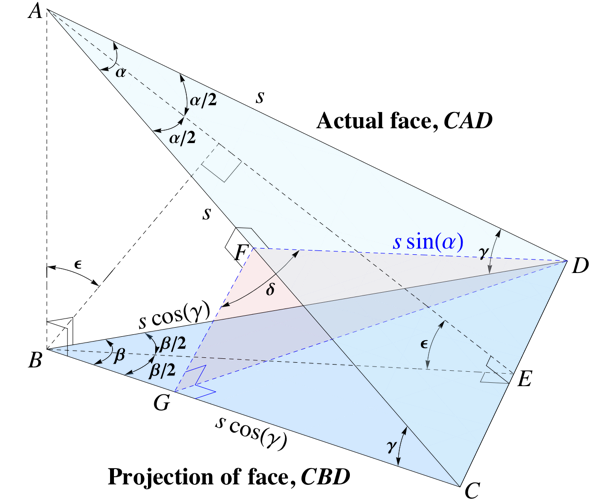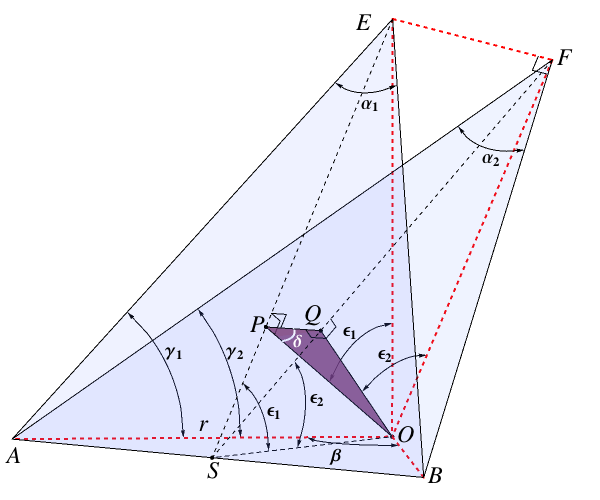# Dihedral Angles

## by Greg Egan

This page derives some simple formulas that are useful for computing dihedral angles in polyhedra.

### Basic formulas

We often start by knowing both the true angle, α, between two edges of a face of a polyhedron, and the angle β that the same two edges appear to make in some projection. For example, every face of a regular icosahedron is an equilateral triangle, with angles of π/3 between the edges — but when we look straight at a vertex of the icosahedron, we see five edges in a projection where the angles between them appear to be 2π/5.To see how we can make use of this kind of information, let’s suppose we have an isoceles triangle with two sides of length s and a known angle α between them (triangle CAD in the diagram). And suppose that this triangle’s projection in some plane is again an isoceles triangle, this time with an angle β between its sides (triangle CBD).

We construct the point E as the midpoint of CD. We find the plane that’s perpendicular to the line segment AC and passes through D, and label the point where this plane cuts AC as F and the point where it cuts BC as G.

Some angles we might then want to know are:

• γ, the angle between the edges of the original triangle and the projection plane.
• ε, the dihedral angle between the original triangle and the projection plane.
• δ, the dihedral angle between the original triangle and the right triangle ABC perpendicular to the projection plane.

If we have a regular polyhedron, the reflection of triangle CAD in the plane of ABC will be an adjacent face of the same polyhedron, and the angle between the two faces will be 2δ.

To find γ, note that we can compute the length EC in two ways: either as s sin ½α from triangle AEC, or as s cos γ sin ½β from triangle BEC. Equating the two we have:

cos γ = sin ½α / sin ½β

To find δ, we use the fact that we can obtain the length GD in two ways: either as s sin α sin δ from triangle FGD, or as s cos γ sin β, from triangle BGD. When we equate these and solve for sin δ, we get:

sin δ = [sin β / sin α] cos γ = [(2 sin ½β cos ½β) / (2 sin ½α cos ½α)] (sin ½α / sin ½β)

which simplifies to:

sin δ = cos ½β / cos ½α

To find ε, we see that:

cos ε = BE / AE = (EC cot ½β) / (EC cot ½α)

This gives us:

cos ε = tan ½α / tan ½β

### Application to Regular Polyhedra

Suppose we have a regular polyhedron in which n faces, all of them regular f-gons, meet at every vertex. If α is the interior angle between edges of an f-gon:

α = (1 – 2/f) π
½α = π/2 – π/f
cos ½α = sin π/f

Looking straight at the vertex, if β is the projected angle between the edges:

β = 2π/n
½β = π/n

The sine of half the internal dihedral angle between the faces of the polyhedron will then be given by our formula from the previous section:

sin δ = cos ½β / cos ½α = (cos π/n) / (sin π/f)

To calculate the full dihedral angle, 2δ, it’s convenient to rewrite this as:

cos 2δ = 1 – 2 sin2 δ = 1 – 2 [(cos π/n) / (sin π/f)]2
Polyhedronnfcos 2δDihedral angle 2δ
Tetrahedron331/370.5288°
Cube34090°
Octahedron43–1/3109.471°
Dodecahedron35–1/√5116.565°
Icosahedron53–(√5)/3138.19°

### Generalisation to Regular PolytopesThe 4-dimensional regular polytope known as the 24-cell has 24 octahedral hyperfaces. At each of its 24 vertices, 6 of these octahedra meet. But the corners of an octahedron (above left) don’t have the correct angles for six of them to fit together without any gaps. What’s needed to do that is the kind of pyramid that can be formed by taking one-sixth of a cube (above right).

Of course, the resolution to this is that the six octahedra that meet at each vertex of a 24-cell don’t all lie in the same 3-dimensional hyperplane, any more than the four triangular faces that meet at each vertex of the octahedron lie in the same 2-dimensional plane. But if we project a view of those four triangles into a plane perpendicular to a line from the centre of the octahedron to the vertex, we see a square divided into four identical triangles. Similarly, if we project a view of the six octahedra that meet at each vertex of the 24-cell into a 3-dimensional hyperplane perpendicular to a line from the centre of the 24-cell to the vertex, we see six identical pyramids of the kind produced by subdividing a cube.

There are analogous situations at the vertices of all the 4-dimensional regular polytopes: the corners of the hyperfaces that come together there will be pyramids with n-sided polygons as their bases, but in the projection of the vertex into a suitable hyperplane there will be shorter pyramids formed by subdividing a “vertex figure” polyhedron with n-sided faces.

The initial known quantities in the problem are:

• α1 = (1 – 2/fF) π, the corner angle of the fF-gon faces of the polytope’s actual hyperfaces;
(in our example, with octahedral hyperfaces, fF = 3 and α1 = π/3)
• α2, the angle between the vertices of the vertex figure polyhedron, subtended at the centre, easily found from the dihedral angles of the dual polyhedron to the vertex figure;
(in our example, the vertex figure is a cube, whose dual is an octahedron, so α2 = π – [dihedral angle for octahedron] = cos–11/3)
• β = 2π/fV, the angle subtended by the sides of the fV-gon faces of the vertex figure;
(in our example, fV = 4 and β = π/2)From the calculations we did in the first section of this page, we have:

cos γ1 = sin ½α1 / sin ½β
cos γ2 = sin ½α2 / sin ½β
cos ε1 = tan ½α1 / tan ½β
cos ε2 = tan ½α2 / tan ½β

In order to find the dihedral angle between hyperfaces of the polytope, we will initially calculate half that angle: δ, the angle between the hyperplane containing the single hyperface we’ve been dealing with (the pyramid with tip E), and the hyperplane containing the tetrahedron ABEF. That tetrahedron contains one face of the hyperface (the triangle ABE), and also the vector EF that is normal to the hyperplane we project into. It’s the four-dimensional analogue of the triangle ABC in our original three-dimensional treatment: the reflection in it of the single hyperface we’re analysing will produce an adjacent hyperface of the same regular polytope.

Now, the heights of the two pyramids are:

OE = r tan γ1
OF = r tan γ2

where r is the radius of the circumscribing circle of their common base. The perpendicular distances from a face of either pyramid to the centre of the base can then by found by using the cosine of the dihedral angle between the base and the face:

OP = OE cos ε1 = r cos ε1 tan γ1
OQ = OF cos ε2 = r cos ε2 tan γ2

The line segment OQ is perpendicular to the entire hyperplane of the tetrahedron ABEF. Why? It’s perpendicular to the face ABF by construction, and it’s perpendicular to EF because EF is normal to the hyperplane of projection that contains the whole projected pyramid, and we constructed OQ to lie within that pyramid.

But the line segment PQ lies within the tetrahedron ABEF, because each endpoint lies on one of its faces. This means OQP is a right triangle with a right angle at Q.

Now, OQ is perpendicular to the face ABE, as is OP by construction, so any vector in the plane of OQP will also be perpendicular to ABE. But the vector that’s normal to the entire unprojected hyperface must be normal to that face, so the hyperface normal must lie in the plane of OQP. What’s more, the hyperface normal must be perpendicular to the line segment OP, which lies within the hyperface. This is enough to tell us that the angle at P in the triangle must be δ, the angle between the hyperface and the tetrahedron ABEF.

So sin δ can be found from the right triangle OQP:

sin δ = OQ / OP
= (cos ε2 tan γ2) / (cos ε1 tan γ1)
= (cos ½α1 / cos ½α2) (sin γ2 / sin γ1)
= (cos ½α1 / cos ½α2) √[(sin2 ½β – sin2 ½α2)/(sin2 ½β – sin2 ½α1)]

The specific values we wish to substitute into this formula are:

½α1 = ½(1 – 2/fF) π = π/2 – π/fF
cos ½α1 = sin π/fF
sin ½α1 = cos π/fF
β = 2π/fV
cos ½α2 = (cos π/fV) / (sin π/dV)

For ½α2, we’re using our formula from the previous section for the dihedral angle for a polyhedron, and applying it to the angle between vertices for the dual polyhedron; one is just π minus the other, so when we take half the angle that exchanges sin and cos. Here we’ve set the number of faces meeting at each vertex to fV, the sides-per-face of the vertex figure, since we’re applying the formula to the dual of the vertex figure. And we’ve introduced a new parameter, dV, which is the number of faces that meet at each vertex in the vertex figure.

This yields a final result of:

sin δ = (cos π/dV) (sin π/fF) / √(sin2 π/fV – cos2 π/fF)

An equivalent formulation is:

cos 2δ = 1 – 2 [(cos π/dV) (sin π/fF)]2 / [sin2 π/fV – cos2 π/fF]
PolytopeHyperfacesfFVertex figuresfVdVcos 2δDihedral angle 2δ
4-simplexTetrahedra3Tetrahedra331/475.5225°
HypercubeCubes4Tetrahedra33090°
Cross-polytopeTetrahedra3Octahedra34–1/2120°
24-cellOctahedra3Cubes43–1/2120°
120-cellDodecahedra5Tetrahedra33–(1 + √5)/4144°
600-cellTetrahedra3Icosahedra35–(1 + 3√5)/8164.478°Science Notes / Dihedral Angles / created Wednesday, 11 April 2012 / revised Saturday, 14 April 2012